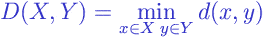# Glossary

The single linkage clustering method (or the nearest neighbor method) is a method of calculating distance between clusters in hierarchical cluster analysis . The linkage function specifying the distance between two clusters is computed as the minimal object-to-object distance, where objectsbelong to the first cluster, objectsbelong to the second cluster. In other words, the distance between two clusters is computed as the distance between the two closest objects in the two clusters.

Mathematically the linkage function – the distancebetween clustersand– is described by the following expression :where

•is the distance between objectsand;
•andare two sets of objects (clusters)

Browse Other Glossary Entries

## Test Yourself

Planning on taking an introductory statistics course, but not sure if you need to start at the beginning? Review the course description for each of our introductory statistics courses and estimate which best matches your level, then take the self test for that course. If you get all or almost all the questions correct, move on and take the next test.

### Data Analytics

Considering becoming adata scientist, customer analyst or our data science certificate program?

Analytics Quiz

Statistics Quiz

### Statistics

Looking at statistics for graduate programs or to enhance your foundational knowledge?

Statistics 1 Quiz

Regression Quiz

Regression Quiz

### Biostatistics

Entering the biostatistics field? Test your skill here.

Biostatistics Quiz

Statistics 2 Quiz

### Stay Informed

Our Blog

Read up on our latest blogs

Certificates

Courses

Find the right course for you